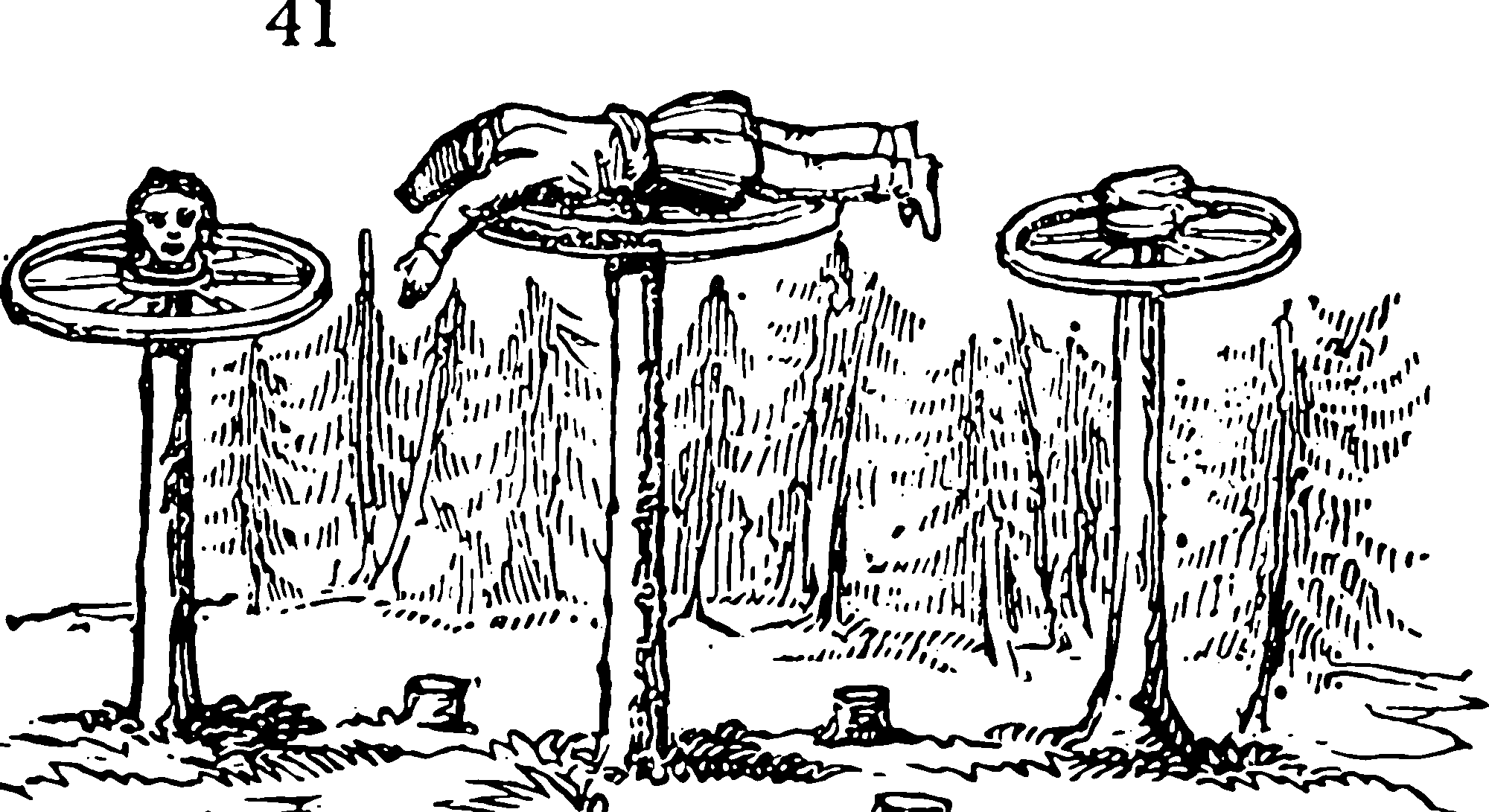# GP inducing variablesA classic trick for factoring GP likelihoods.

Sparse Gaussian processes are ones where you approximate the target posterior by summarising the data with a short list of inducing points that have nearly the same similar posterior density, by some metric. These have been invented in various forms by various people, but most of the differences we can ignore. Based on citations, thethe Right Way is the version of Titsias (2009), which we use in all the subsequent things as a building-block.

An explanation, based on Hensman, Fusi, and Lawrence (2013) summarizing Titsias (2009):

We consider an output vector $$\mathbf{y},$$ where each entry $$y_{i}$$ is a noisy observation of the function $$f,$$ i.e. $$y_{i}=f(\mathbf{x}_{i})+\varepsilon_{i}$$ for all the points $$\mathbf{X}=\left\{\mathbf{x}_{i}\right\}_{i=1}^{n}.$$ We assume the noises $$\varepsilon_{i}\sim\mathcal{N}(0,1/\beta)$$ to be independent. We introduce a Gaussian process prior over $$f(\cdot).$$ Now let the vector $$\mathbf{f}=\{f(x_{i})\}_{i=1}^{n}$$ contain values of the function evaluated at the inputs. We introduce a set of inducing variables, defined similarly: let the vector $$\mathbf{u}=\{f(\mathbf{z}_{i})\}_{i=1}^{m}$$. Standard properties of Gaussian processes allow us to write \begin{aligned} p(\mathbf{y} \mid \mathbf{f}) &=\mathcal{N}\left(\mathbf{y} \mid \mathbf{f}, \beta^{-1} \mathbf{I}\right) \\ p(\mathbf{f} \mid \mathbf{u}) &=\mathcal{N}\left(\mathbf{f} \mid \mathbf{K}_{n m} \mathbf{K}_{m m}^{-1} \mathbf{u}, \tilde{\mathbf{K}}\right) \\ p(\mathbf{u}) &=\mathcal{N}\left(\mathbf{u} \mid \mathbf{0}, \mathbf{K}_{m m}\right) \end{aligned} where $$\mathbf{K}_{m m}$$ is the covariance function evaluated between all the inducing points and $$\mathbf{K}_{n m}$$ is the covariance function between all inducing points and training points and we have defined with $$\tilde{\mathbf{K}}=\mathbf{K}_{n n}-$$ $$\mathbf{K}_{n m} \mathbf{K}_{m m}^{-1} \mathbf{K}_{m n}$$

Quoting them:

We first apply Jensen’s inequality on the conditional probability $$p(\mathbf{y} \mid \mathbf{u})$$ \begin{aligned} \log p(\mathbf{y} \mid \mathbf{u}) &=\log \langle p(\mathbf{y} \mid \mathbf{f})\rangle_{p(\mathbf{f} \mid \mathbf{u})} \\ & \geq\langle\log p(\mathbf{y} \mid \mathbf{f})\rangle_{p(\mathbf{f} \mid \mathbf{u})} \triangleq \mathcal{L}_{1} \end{aligned} where $$\langle\cdot\rangle_{p(x)}$$ denotes an expectation under $$p(x).$$ For Gaussian noise taking the expectation inside the log is tractable, but it results in an expression containing $$\mathbf{K}_{n n}^{-1},$$ which has a computational complexity of $$\mathcal{O}\left(n^{3}\right).$$ Bringing the expectation outside the log gives a lower bound, $$\mathcal{L}_{1},$$ which can be computed with has complexity $$\mathcal{O}\left(m^{3}\right).$$ Further, when $$p(\mathbf{y} \mid \mathbf{f})$$ factorises across the data, $p(\mathbf{y} \mid \mathbf{f})=\prod_{i=1}^{n} p\left(y_{i} \mid f_{i}\right)$ then this lower bound can be shown to be separable across y giving $\exp \left(\mathcal{L}_{1}\right)=\prod_{i=1}^{n} \mathcal{N}\left(y_{i} \mid \mu_{i}, \beta^{-1}\right) \exp \left(-\frac{1}{2} \beta \tilde{k}_{i, i}\right)$ where $$\boldsymbol{\mu}=\mathbf{K}_{n m} \mathbf{K}_{m m}^{-1} \mathbf{u}$$ and $$\tilde{k}_{i, i}$$ is the $$i$$ th diagonal element of $$\widetilde{\mathbf{K}}$$. Note that the difference between our bound and the original log likelihood is given by the Kullback Leibler (KL) divergence between the posterior over the mapping function given the data and the inducing variables and the posterior of the mapping function given the inducing variables only, $\mathrm{KL}(p(\mathbf{f} \mid \mathbf{u}) \| p(\mathbf{f} \mid \mathbf{u}, \mathbf{y}))$ This KL divergence is minimized when there are $$m=n$$ inducing variables and they are placed at the training data locations. This means that $$\mathbf{u}=\mathbf{f}$$, $$\mathbf{K}_{m m}=\mathbf{K}_{n m}=\mathbf{K}_{n n}$$ meaning that $$\tilde{\mathbf{K}}=\mathbf{0} .$$ In this case we recover $$\exp \left(\mathcal{L}_{1}\right)=p(\mathbf{y} \mid \mathbf{f})$$ and the bound becomes equality because $$p(\mathbf{f} \mid \mathbf{u})$$ is degenerate. However, since $$m=n$$ and that there would be no computational or storage advantage from the representation. When $$m<n$$ the bound can be maximised with respect to $$\mathbf{Z}$$ (which are variational parameters). This minimises the KL divergence and ensures that $$\mathbf{Z}$$ are distributed amongst the training data $$\mathbf{X}$$ such that all $$\tilde{k}_{i, i}$$ are small. In practice this means that the expectations in (1) are only taken across a narrow domain ($$\tilde{k}_{i, i}$$ is the marginal variance of $$p\left(f_{i} \mid \mathbf{u}\right)$$ ), keeping Jensen’s bound tight.

…we recover the bound of Titsias (2009) by marginalising the inducing variables, \begin{aligned} \log p(\mathbf{y} \mid \mathbf{X}) &=\log \int p(\mathbf{y} \mid \mathbf{u}) p(\mathbf{u}) \mathrm{d} \mathbf{u} \\ & \geq \log \int \exp \left\{\mathcal{L}_{1}\right\} p(\mathbf{u}) \mathrm{d} \mathbf{u} \triangleq \mathcal{L}_{2} \end{aligned} which with some linear algebraic manipulation leads to $\mathcal{L}_{2}=\log \mathcal{N}\left(\mathbf{y} \mid \mathbf{0}, \mathbf{K}_{n m} \mathbf{K}_{m m}^{-1} \mathbf{K}_{m n}+\beta^{-1} \mathbf{I}\right)-\frac{1}{2} \beta \operatorname{tr}(\widetilde{\mathbf{K}})$ matching the result of Titsias, with the implicit approximating distribution $$q(\mathbf{u})$$ having precision $\mathbf{\Lambda}=\beta \mathbf{K}_{m m}^{-1} \mathbf{K}_{m n} \mathbf{K}_{n m} \mathbf{K}_{m m}^{-1}+\mathbf{K}_{m m}^{-1}$ and mean $\hat{\mathbf{u}}=\beta \mathbf{\Lambda}^{-1} \mathbf{K}_{m m}^{-1} \mathbf{K}_{m n} \mathbf{y}$

### No comments yet. Why not leave one?

GitHub-flavored Markdown & a sane subset of HTML is supported.